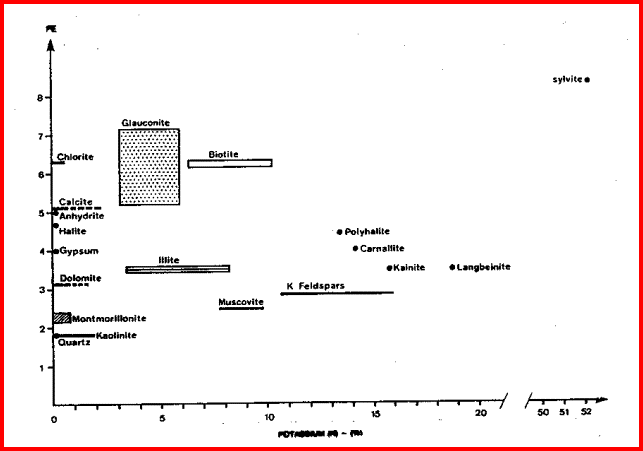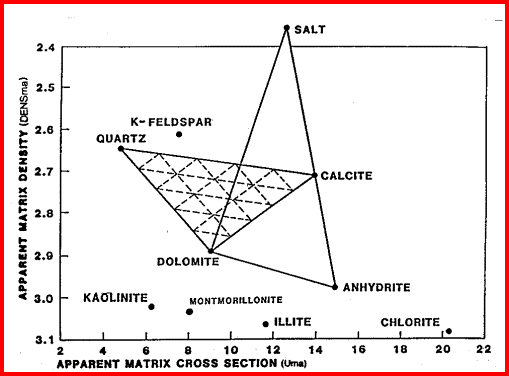CROSSPLOTS FOR CLAY TYPING

Clay typing from well log data is usually accomplished by using crossplots of  potassium and thorium data from the gamma ray spectral log and the photo electric effect. If the shale is somewhat silty, the non-clay minerals will skew the results, requiring a detailed multi-mineral model calculation. Density, neutron, and PE data will also help sort out clay types.

 Th/K Ratio High Kaolinite Chlorite Montemorillonite Illite Mica Glauconite Feldspar Low Potassium evaporateTHORIUM / POTASSIUM CROSSPLOTS
The thorium/potassium ratio method of visually identifying clay-type can be used with the aid of the table at the right, or with the calculator shown below..

Thorium is recorded in parts per million (ppm) and potassium in percent (%), and the Th/K ratio is therefore in units of (Th-ppm) / (K-%). For example if THOR = 9 ppm and POTA = 3.0%, the ratio is 9 / 3 = 3.0. This is in the mid-range of these ratios and the interpretation could be illite clay or mica or both in this

shale.

CLAY TYPE from Gamma Ray Spectral Data
Enter Potasium value POTA - %:
Enter Thorium value THOR - ppm:
Enter values above then press for result:

This ratio is illustrated graphically below.PE vs Th/K Ratio Crossplot for Clay Typing. Thorium is recorded in ppm and Potassium in % by
volume, so units on X axis are ppm / %. The PE value helps distinguish between minerals with similar Th/K ratios. Note that clay and mica minerals do not hve unique values but ranges of values for Th/K and some have a wide rangee for PE as well.PE / POTASSIUM CROSSPLOTS
This crossplot distributes the clay minerals in the X-Y space somewhat differentky than the PE vs Th/K crossplot. It may give better resolution in some mineral combinations. Two and three mineral models can be developed for various mineral mixtures.PE vs Potassium Crossplot for  Clay Typing. If data points fall to the left of the clay points, the
data is from a silty shale or calcareous shale. Units on X axis are Potassium % by volume.Ush - Apparent Matrix Photoelectric Cross Section of Shale
The matrix density (DFNSma) versus matrix cross section (Uma) crossplot is usually used to solve a 3-mineral model, but it can be used for clay typing by plotting data from shale intervals. If data is in porosity units, convert PHIDSH to density with equation 2. Calculate Ush from equation 3. Plot DENSSH on vertical axis and Ush on horizontal axis.

1: PHIt = (PHINSH + PHIDSH) / 2
2: DENSSH =  PHIDSH * KD1+ (1 - PHIDSH) * KD2
3: USH = PESH * DENSSH / PHIt

Where:
KD1 = 1.00,  KD2 = 2.65 for Sandstone scale or 2.71 for Limestone scale
DENSSH = density log reading in shale (gm/cc)
PESH = effective photoelectric cross section in shale (barns/cm3)
PHINSH = neutron porosity in shale (fractional)
PHIDSH = density porosity in shale (fractional)
PHINSH = neutron porosity in shale (fractional)
USH = photoelectric absorption of shale (barns/cm3)Matrix Density vs Matrix Cross Section Crossplot for Lithology. If plotted data points fall
above the clay points, the data is from a silty shale or a calcareous shale.

Page Views ---- Since 01 Jan 2015
Copyright 2023 by Accessible Petrophysics Ltd.
CPH Logo, "CPH", "CPH Gold Member", "CPH Platinum Member", "Crain's Rules", "Meta/Log", "Computer-Ready-Math", "Petro/Fusion Scripts" are Trademarks of the Author